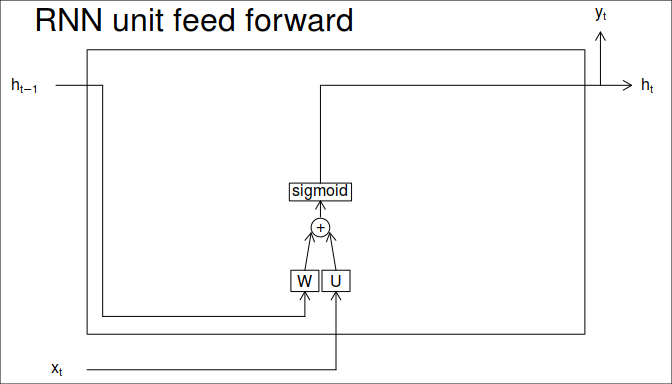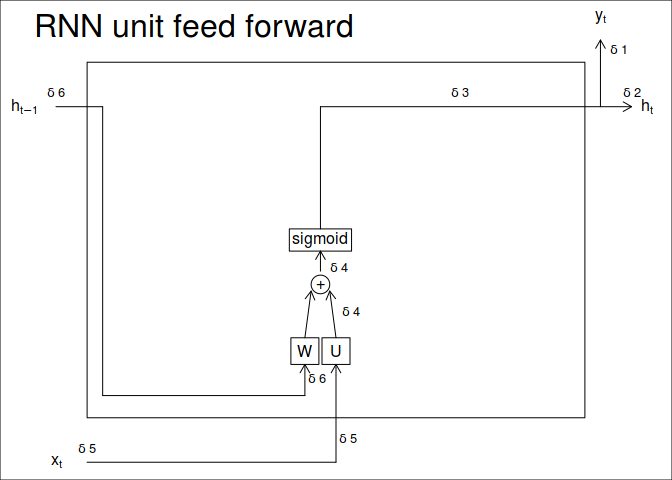# Feed forward pass$h_t = y_t = sigmoid(h_{t-1} * W + x_t * U)$

# Back propagation passTo perform the BPTT with a RNN unit, we have the eror comming from the top layer ($$\delta 1$$), the future hidden state ($$\delta 2$$). Also, we have stored during the feed forward the states at each step of the feeding. In the case of the future layer, this error is just set to zero if not calculated yet. For convention, $$\cdot$$ correspond to point wise multiplication, while $$*$$ correspond to matrix multiplication.

The rules on how to back prpagate come from this post.

$\delta 3 = \delta 1 + \delta 2$

$\delta 4 = \delta 3 \cdot sigmoid'(h_t)$

$\delta 5 = \delta 4 * W^T$ $\delta 6 = \delta 4 * U^T$

The error $$\delta 5$$ and $$\delta 6$$ are used for the next layers. Once all those errors are available, it is possible to calculate the weight update.

$\delta W = \delta W + h_{t-1}^T * \delta 4$

$\delta U = \delta U + x_{t}^T * \delta 5$

This should be according to the linked post but in reality, we did it as follow:

$\delta 5 = \delta 6 = ((\delta 2 * W^T) + (\delta 1 * U^T)) * sigmoid'(h_t)$

$\delta U = \delta U + x_{t}^T * \delta 1$

$\delta W = \delta W + h_{t-1}^T * \delta 2$